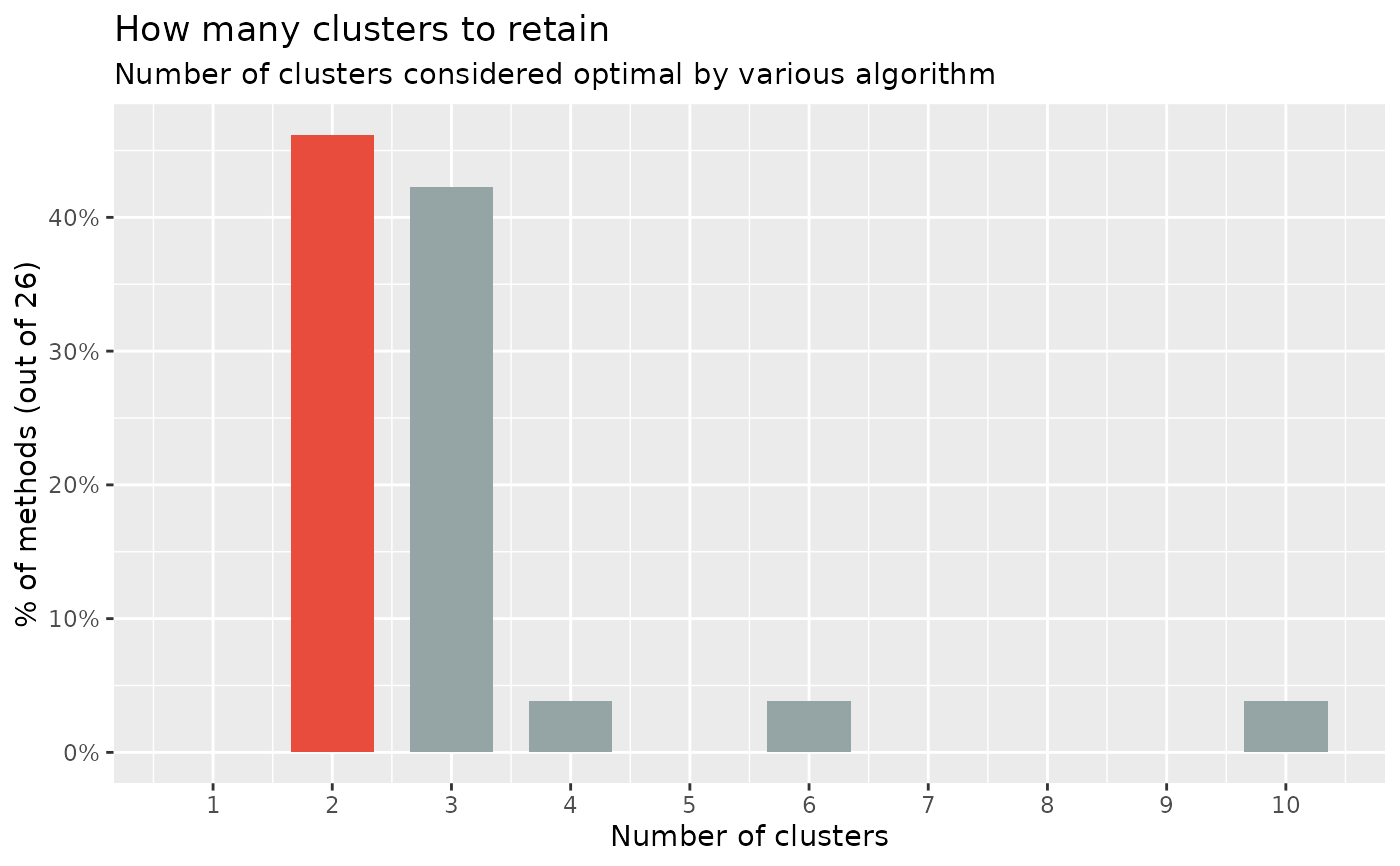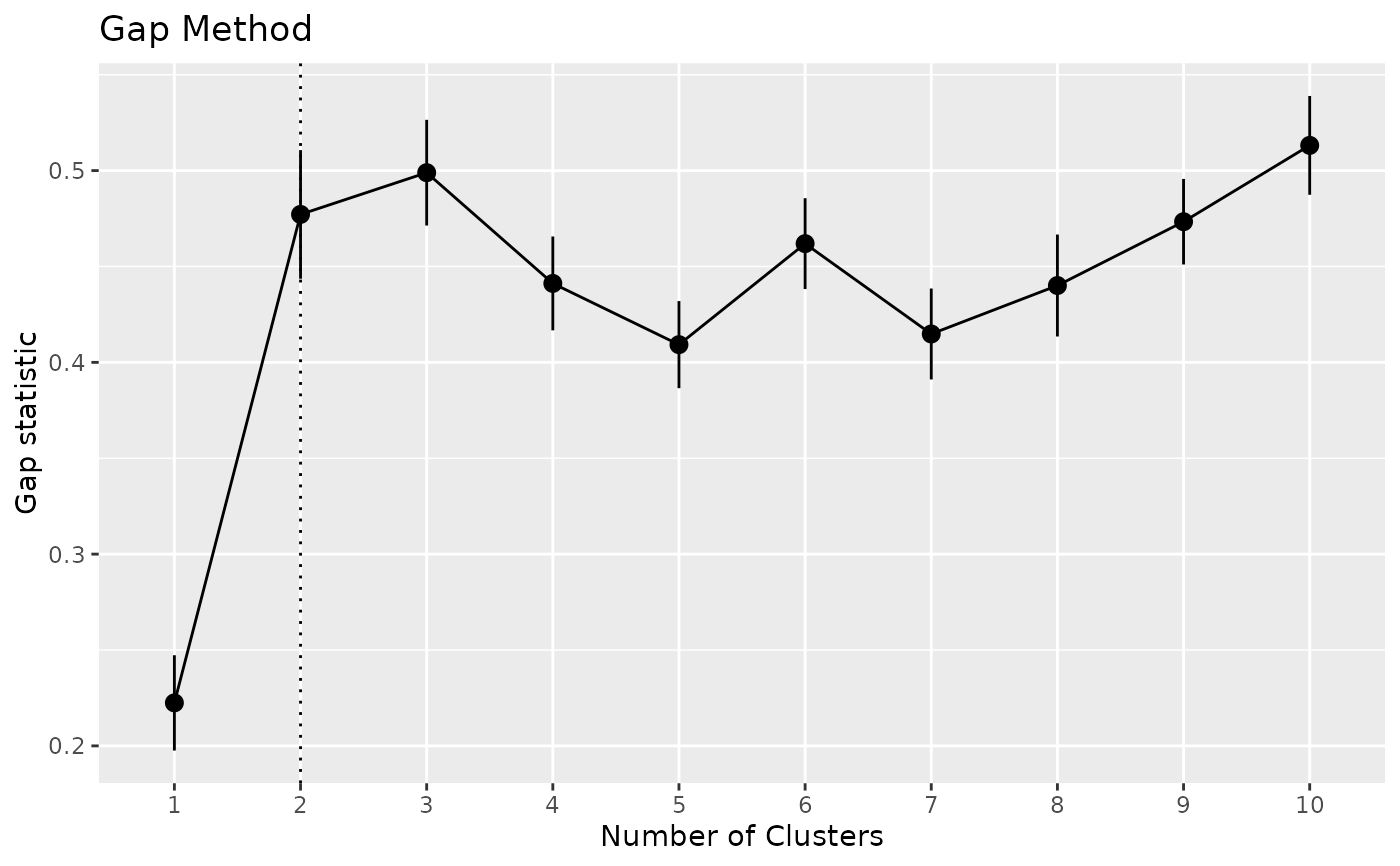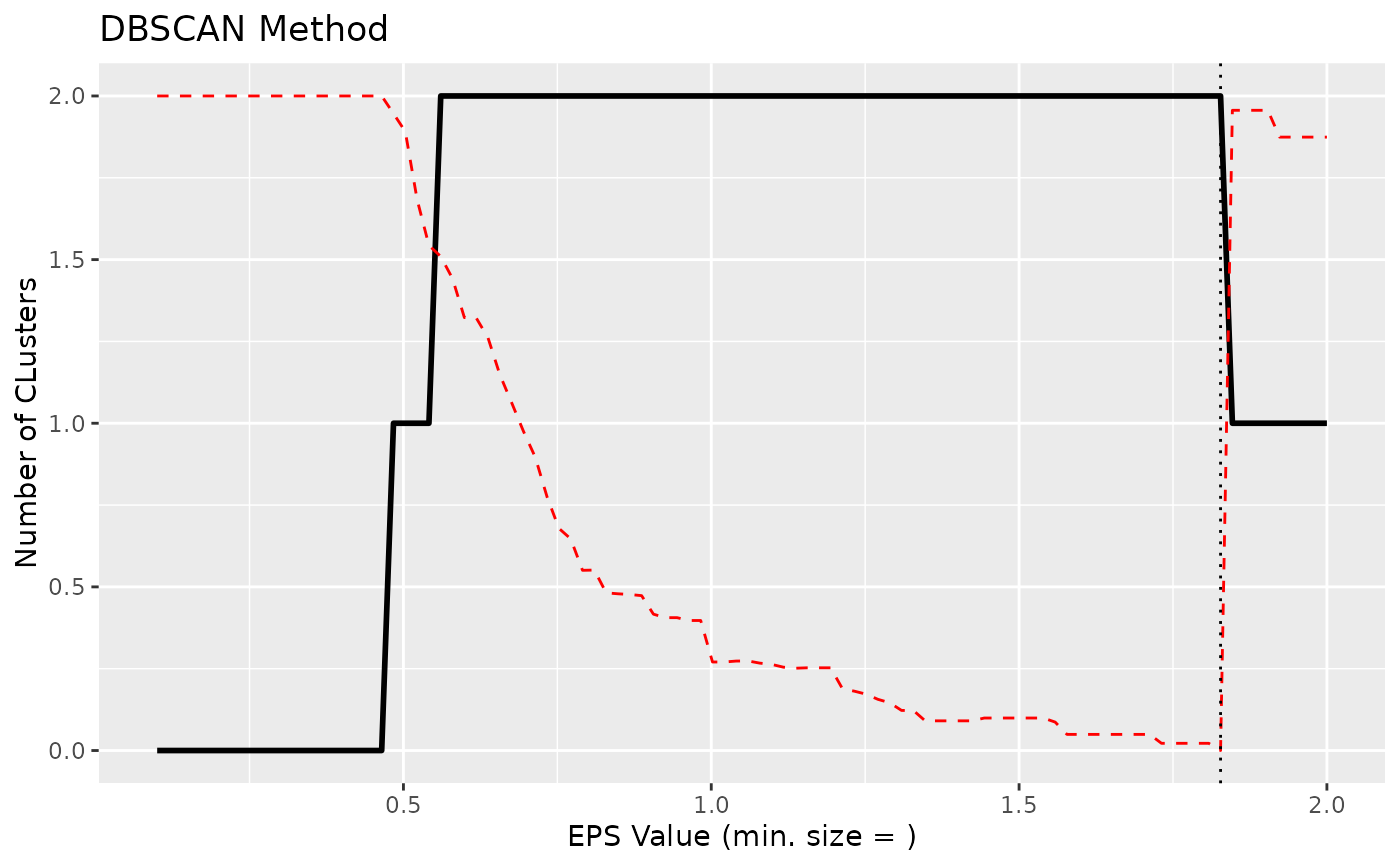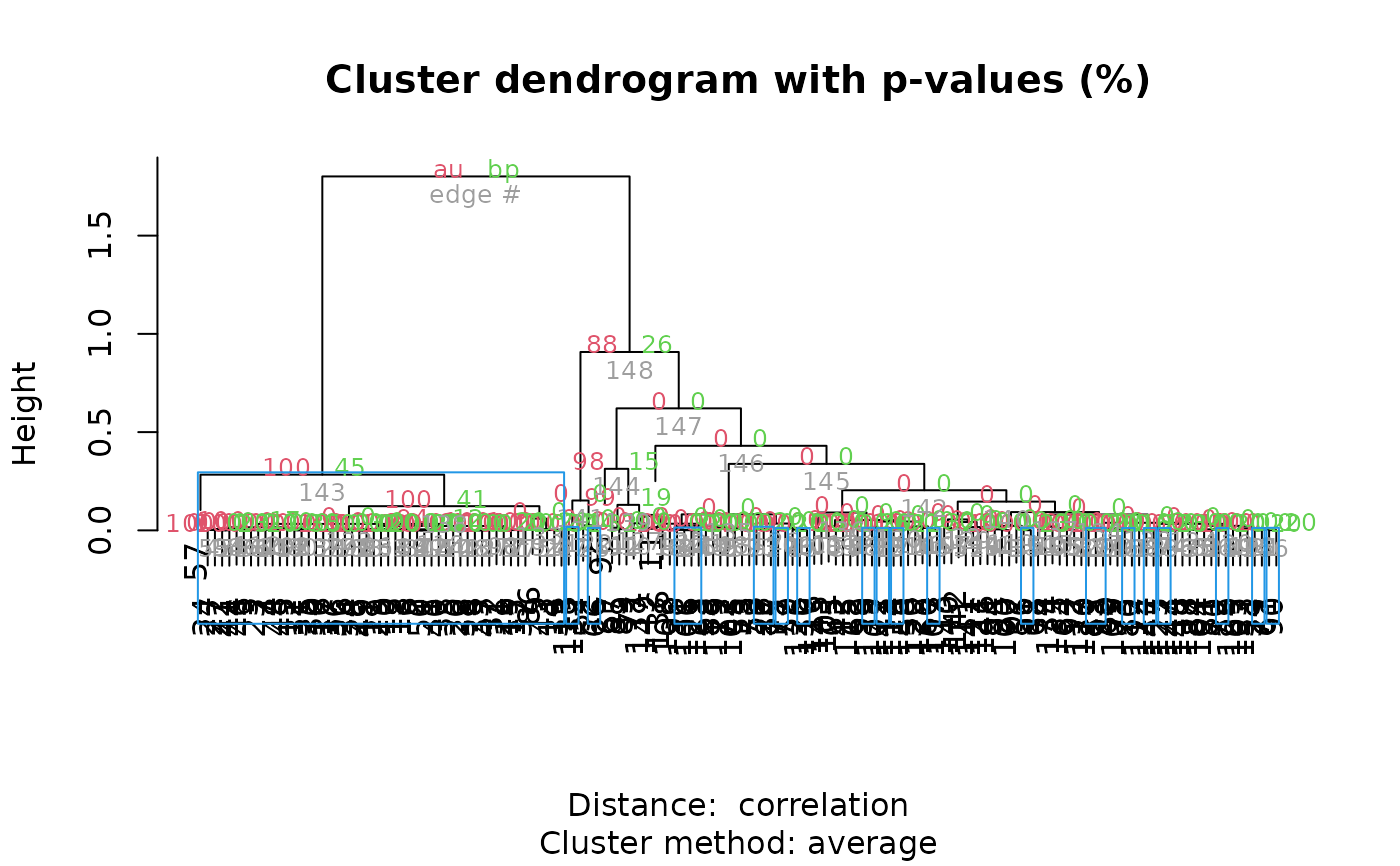Similarly to n_factors() for factor / principal component analysis, n_clusters() is the main function to find out the optimal numbers of clusters present in the data based on the maximum consensus of a large number of methods.

Essentially, there exist many methods to determine the optimal number of clusters, each with pros and cons, benefits and limitations. The main n_clusters function proposes to run all of them, and find out the number of clusters that is suggested by the majority of methods (in case of ties, it will select the most parsimonious solution with fewer clusters).

Note that we also implement some specific, commonly used methods, like the Elbow or the Gap method, with their own visualization functionalities. See the examples below for more details.

## Usage

n_clusters(
x,
standardize = TRUE,
include_factors = FALSE,
package = c("easystats", "NbClust", "mclust"),
fast = TRUE,
nbclust_method = "kmeans",
n_max = 10,
...
)

n_clusters_elbow(
x,
standardize = TRUE,
include_factors = FALSE,
clustering_function = stats::kmeans,
n_max = 10,
...
)

n_clusters_gap(
x,
standardize = TRUE,
include_factors = FALSE,
clustering_function = stats::kmeans,
n_max = 10,
gap_method = "firstSEmax",
...
)

n_clusters_silhouette(
x,
standardize = TRUE,
include_factors = FALSE,
clustering_function = stats::kmeans,
n_max = 10,
...
)

n_clusters_dbscan(
x,
standardize = TRUE,
include_factors = FALSE,
method = c("kNN", "SS"),
min_size = 0.1,
eps_n = 50,
eps_range = c(0.1, 3),
...
)

n_clusters_hclust(
x,
standardize = TRUE,
include_factors = FALSE,
distance_method = "correlation",
hclust_method = "average",
ci = 0.95,
iterations = 100,
...
)

## Arguments

x

A data frame.

standardize

Standardize the dataframe before clustering (default).

include_factors

Logical, if TRUE, factors are converted to numerical values in order to be included in the data for determining the number of clusters. By default, factors are removed, because most methods that determine the number of clusters need numeric input only.

package

Package from which methods are to be called to determine the number of clusters. Can be "all" or a vector containing "easystats", "NbClust", "mclust", and "M3C".

fast

If FALSE, will compute 4 more indices (sets index = "allong" in NbClust). This has been deactivated by default as it is computationally heavy.

nbclust_method

The clustering method (passed to NbClust::NbClust() as method).

n_max

Maximal number of clusters to test.

...

Arguments passed to or from other methods. For instance, when bootstrap = TRUE, arguments like type or parallel are passed down to bootstrap_model().

clustering_function, gap_method

Other arguments passed to other functions. clustering_function is used by fviz_nbclust() and can be kmeans, cluster::pam, cluster::clara, cluster::fanny, and more. gap_method is used by cluster::maxSE to extract the optimal numbers of clusters (see its method argument).

method, min_size, eps_n, eps_range

Arguments for DBSCAN algorithm.

distance_method

The distance method (passed to dist()). Used by algorithms relying on the distance matrix, such as hclust or dbscan.

hclust_method

The hierarchical clustering method (passed to hclust()).

ci

Confidence Interval (CI) level. Default to 0.95 (95%).

iterations

The number of bootstrap replicates. This only apply in the case of bootstrapped frequentist models.

## Note

There is also a plot()-method implemented in the see-package.

## Examples

# \donttest{
library(parameters)

# The main 'n_clusters' function ===============================
if (require("mclust", quietly = TRUE) && require("NbClust", quietly = TRUE) &&
require("cluster", quietly = TRUE) && require("see", quietly = TRUE)) {
n <- n_clusters(iris[, 1:4], package = c("NbClust", "mclust")) # package can be "all"
n
summary(n)
as.data.frame(n) # Duration is the time elapsed for each method in seconds
plot(n)

# The following runs all the method but it significantly slower
# n_clusters(iris[1:4], standardize = FALSE, package = "all", fast = FALSE)
}# }
# \donttest{
x <- n_clusters_elbow(iris[1:4])
x
#> The Elbow method, that aims at minimizing the total intra-cluster variation (i.e., the total within-cluster sum of square), suggests that the optimal number of clusters is 2.
as.data.frame(x)
#>    n_Clusters       WSS
#> 1           1 596.00000
#> 2           2 220.87929
#> 3           3 138.88836
#> 4           4 113.64981
#> 5           5  90.22782
#> 6           6  95.25396
#> 7           7  72.75296
#> 8           8  64.61603
#> 9           9  59.48502
#> 10         10  59.10865
plot(x)# }
# \donttest{
#
# Gap method --------------------
if (require("see", quietly = TRUE) &&
require("cluster", quietly = TRUE) &&
require("factoextra", quietly = TRUE)) {
x <- n_clusters_gap(iris[1:4])
x
as.data.frame(x)
plot(x)
}# }
# \donttest{
#
# Silhouette method --------------------------
if (require("factoextra", quietly = TRUE)) {
x <- n_clusters_silhouette(iris[1:4])
x
as.data.frame(x)
plot(x)
}# }
# \donttest{
#
if (require("dbscan", quietly = TRUE)) {
# DBSCAN method -------------------------
# NOTE: This actually primarily estimates the 'eps' parameter, the number of
# clusters is a side effect (it's the number of clusters corresponding to
# this 'optimal' EPS parameter).
x <- n_clusters_dbscan(iris[1:4], method = "kNN", min_size = 0.05) # 5 percent
x
plot(x)

x <- n_clusters_dbscan(iris[1:4], method = "SS", eps_n = 100, eps_range = c(0.1, 2))
x
plot(x)
}# }
# \donttest{
#
# hclust method -------------------------------
if (require("pvclust", quietly = TRUE)) {
# iterations should be higher for real analyses
x <- n_clusters_hclust(iris[1:4], iterations = 50, ci = 0.90)
x
head(as.data.frame(x), n = 10) # Print 10 first rows
plot(x)
}# }